## 6.3  Many Degrees of Freedom

Other problems are encountered in applying perturbation theory to systems with more than a single degree of freedom. Consider a Hamiltonian of the form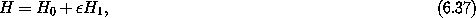where H0 depends only on the momenta and so is solvable. We assume that the Hamiltonian has no explicit time dependence. We further assume that the coordinates are all angles and that H1 is a multiply periodic function of the coordinates.

Carrying out a Lie transformation with generator W produces the Hamiltonian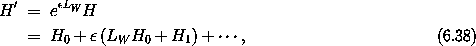as before. The condition that the order-terms are eliminated is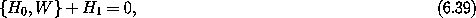a linear partial differential equation. By assumption, the Hamiltonian H0 depends only on the momenta. We define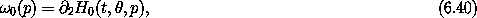the tuple of frequencies of the unperturbed system. The condition on W is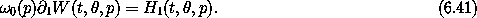As H1 is a multiply-periodic function of the coordinates, we can write it as a Poisson series:1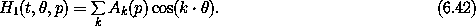Similarly, we assume W can be written as a Poisson series: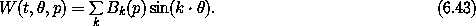Substituting these into the condition that order-terms are eliminated, we find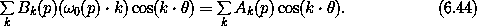The cosines are orthogonal so each term must be individually zero. We deduce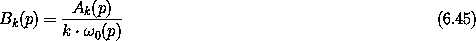and that the required Lie generator is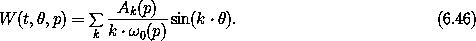There are a couple of problems. First, if A0 is nonzero then the expression for B0 involves a division by zero. So the expression for B0 is not correct. The problem is that the corresponding term in H1 does not involve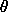. So the integration for B0 should introduce linear terms in. But this is the same situation that led to the secular terms in the perturbation approximation to the pendulum. Having learned our lesson there, we avoid the secular terms by adjoining this term to the solvable Hamiltonian and excluding k = 0 from the sum for W. We have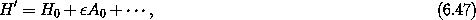and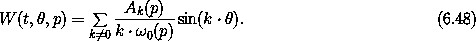Another problem is that there are many opportunities for small denominators that would make the perturbation large and therefore not a perturbation. As we saw in the perturbation approximation for the pendulum in terms of the rotor, we must exclude certain regions from the domain of applicability of the perturbation approximation. These excluded regions are associated with commensurabilities among the frequencies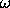0(p). Consider the phase-space transformation of the coordinatesSo we must exclude from the domain of applicability all regions for which the coefficients are large. If the second term dominates, the excluded regions satisfy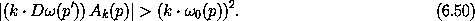Considering the fact that for any tuple of frequencies0(p') we can find a tuple of integers k such that k ·(p') is arbitrarily small, this problem of small divisors looks very serious.

However, the problem, though serious, is not as bad as it may appear, for a couple of reasons. First, it may be that Ak ne 0 only for certain k. In this case, only the regions for these terms are excluded from the domain of applicability. Second, for analytic functions the magnitude of Ak decreases strongly with the size of k (see ):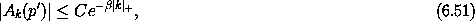for some positive ß and C, and where | k |+ = | k0 | + | k1 | + ··· . At any stage of a perturbation approximation we can limit consideration to just those terms that are larger than a specified magnitude. The size of the excluded region corresponding to a term is of order square root of |Ak(p')| and the inequality (6.51) shows that |Ak(p')| decreases exponentially with the order of the term.

### 6.3.1  Driven Pendulum as a Perturbed Rotor

More concretely, consider the periodically driven pendulum. We will develop approximate solutions for the driven pendulum as a perturbed rotor.

We use the Hamiltonian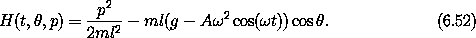We can remove the explicit time dependence by going to the extended phase space. The Hamiltonian is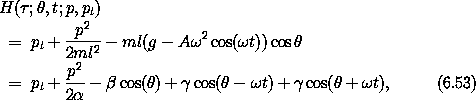with the constants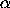= ml2, ß = mlg, and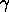= (1/2) m l A2 .

With the intent to approximate the driven pendulum as a perturbed rotor, we choose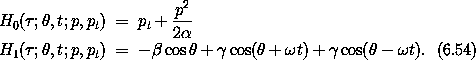Notice that the perturbation H1 has only three terms in its Poisson series, so in the first perturbation step only three regions will be excluded from the domain of applicability. The perturbation H1 is particularly simple: it has only three terms, and the coefficients are constants.

The Lie series generator that eliminates the terms in H1 to first order in, satisfying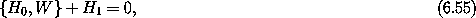is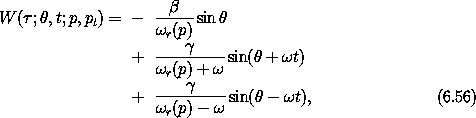wherer(p) =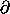2,0 H0(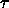;, t; p, pt) = p/is the unperturbed rotor frequency.

The resulting approximate solution has three regions in which there are small denominators, and so three regions that are excluded from applicability of the perturbative solution. Regions of phase space for whichr(p) is near 0,, and -are excluded. Away from these regions the perturbative solution works well, just as in the rotor approximation for the pendulum. Unfortunately, some of the more interesting regions of the phase space of the driven pendulum are excluded: the region in which we find the remnant of the undriven pendulum is excluded, as are the two resonance regions in which the rotation of the pendulum is synchronous with the drive. We need to develop methods for approximating these regions.

1 In general, we need to include sine terms as well, but the cosine expansion is enough for this illustration.